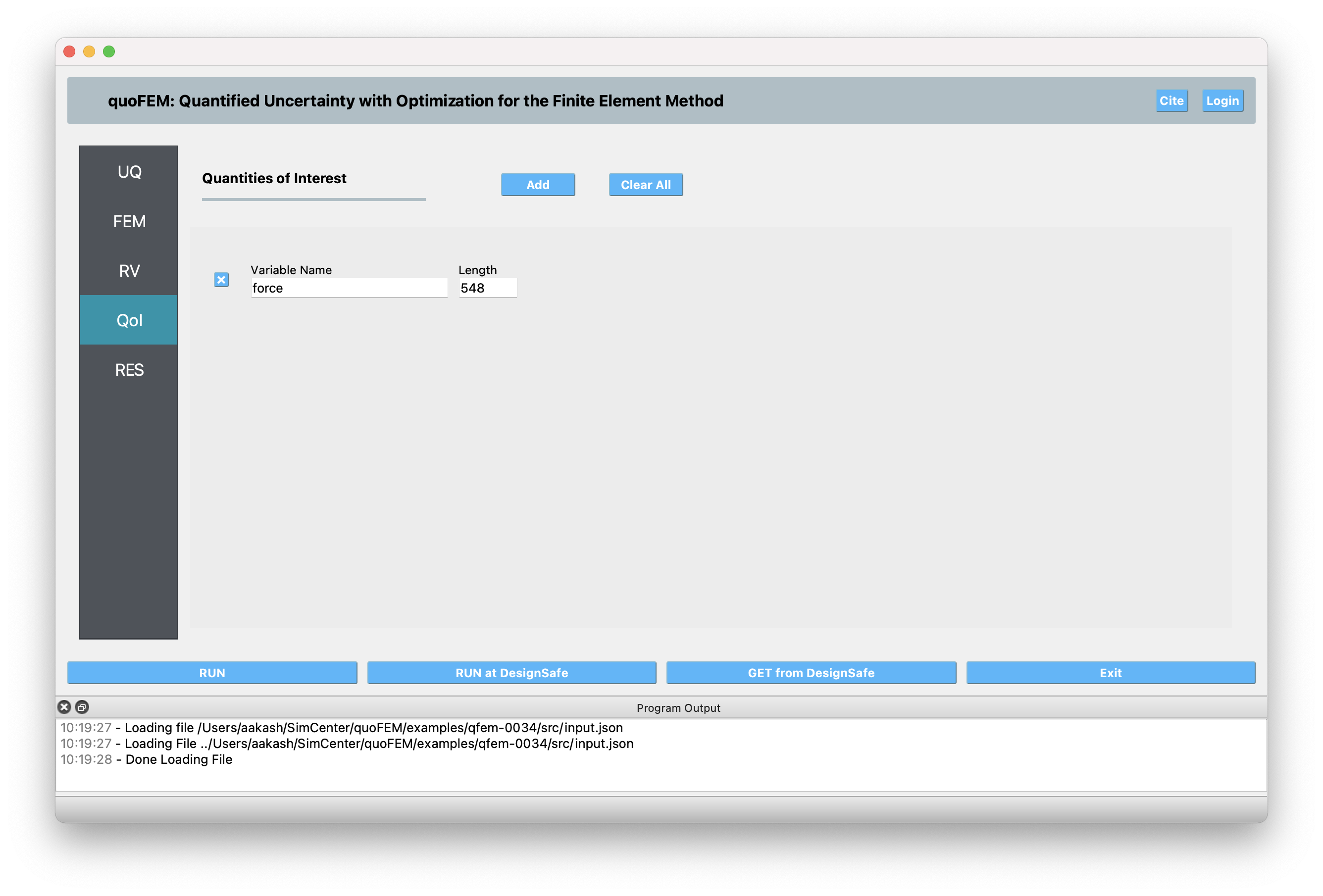# 3.4. QoI: Quantities of Interest¶

The QoI tab is where the user specifies the variable names associated with the FEM (model) simulation results. The user must provide variable names and specify their lengths. The result quantity might be a scalar (i.e. of length 1) or a vector/field quantity (i.e., of length > 1). Having vector/field variables can be convenient when user is interested in high-dimensional output. In most of the algorithms supported in quoFEM, as long as the sum of the lengths match the number of output produced by the model (i.e. number of entries written in `results.out`), resulting UQ output will be the same. However in the following methods, the results/display may be different if a vector/field variable is introduced instead of multiple scalar variables.

A new response quantity is added by the user selecting the Add button. Quantities can be removed by clicking the x button in front of each variable name.Fig. 3.4.1 Input for the QoI tab.

For example, in Fig. 3.4.1, the user has defined three response quantities, `Node_2_Disp_2`, `Node_4_Disp_1` and `stress_time_history`. Since the total length of QoIs is 344, quoFEM expects to 344 values in the `results.out` as the model output. The first two quantities corresponds to `Node_2_Disp_2` and `Node_4_Disp_1`, and the following 342 quantities should be `stress_time_history`. Alternatively, instead of having one vector variable `stress_time_history`, the user may want to divide this into multiple variables with a smaller length. The analysis will run successfully as long as the sum of length values is equal to 344.

If a postprocessing Python script has been provided in the FEM tab, it will be passed these three names as arguments.

Warning

1. There can be no spaces in variable names, underscores are permitted.

2. Names cannot begin with a numeral, i.e. 0,1,2,3,4,5,6,7,8,9.

3. Names cannot be duplicated.